# Formula/Ratio Summary methodsHi Colleagues, Master Anaplanners.

I've recently bumped in some complex calculations where I had to use different logic for different levels of hierarchy, most I've achieved through Ratio summary method, but there's one I cannot get it work. Hope I can get some ideas or out of the box thinking from you:

- NSV/KG is a calculated line item with ratio summary method - I need it's total category level in order to be used in another line item calculation (screenshot 2 for exemplification) - going to use a formula summary method.

- For this to be achieved, I cannot use lookup, but instead to calculate total category in another line item, that will apply to all skus.

- Yes, I am able to pull out total category with formula summary method, but then when I want to apply it to each sku I am forced to use lookup, therefore summary method cannot be formula for NSV category, hence my dilema. (down below I have used Average just to validate monthly calculation for subsequent flows).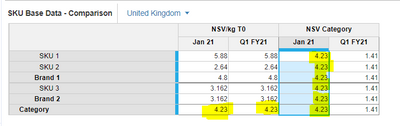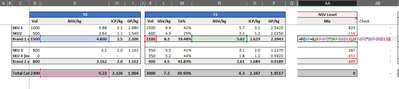Tagged:

•Hi Alexandru,

Not sure if I fully understood but why not split out the NSV Category into a line item which does not have formula summary and has only:

NSV/KG[LOOKUP: List.Parent]

Call this line item as NSV Category Staging. In this Staging line item if you need the same value as the SKU at the Brand and Category level, simply use the Average summary (value 4.23 in your screenshot)

Then in your NSV Category line item use NSV Category Staging as a direct reference and you should be able to set NSV Category as formula summary

Let me know if that works!

•Thanks @anirudh for your reply. That's exactly my approach at the moment, I have it averaged at parent/category levels, but then when we are looking at time dimension, time summary is not correct. I want quarter totals of my new line item to be equal with category totals from NSV/KG - I could only achieve it through formula summary method, but I cannot make a direct refference on NSV/KG if I use lookup.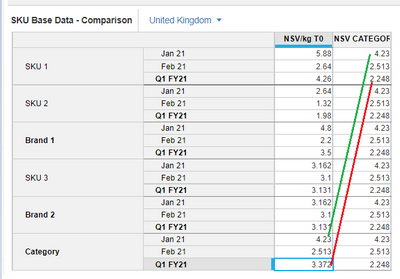•In the new line why don't you Keep Summary as Ratio for Time dimension. For that you may need two additional line items

Numerator - Bring the Quarter totals at category level in this line. You will have to keep the summary as Average since you will be using two LOOKUPs here.

Denominator - Hard Code it to 1 (Keeping summary as Formula)

See screenshot if this helps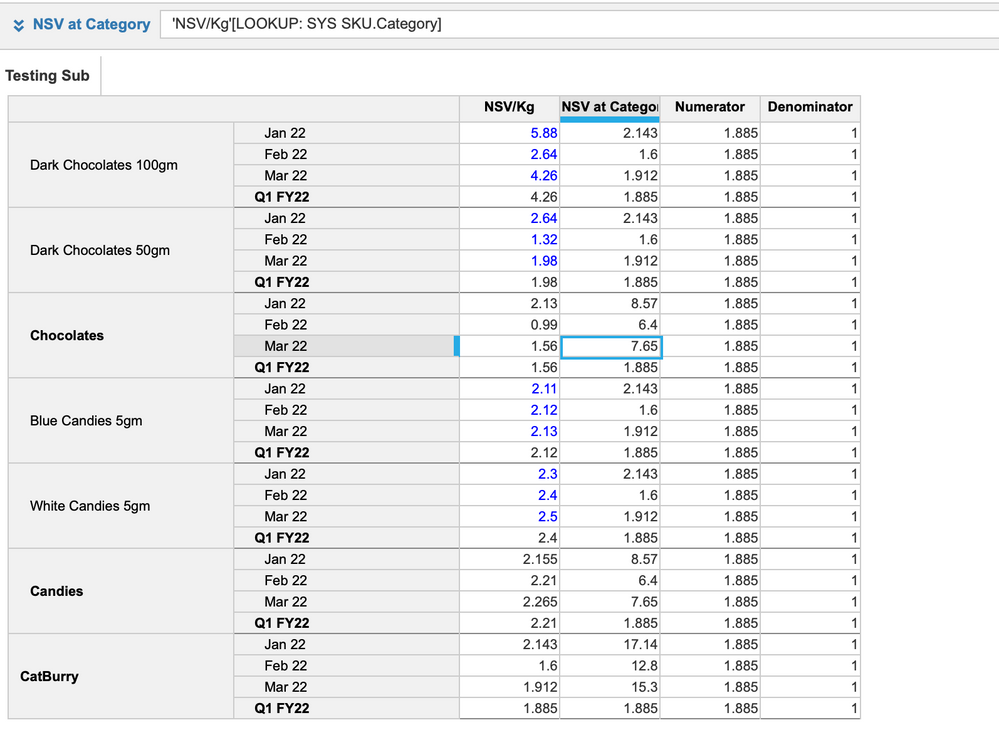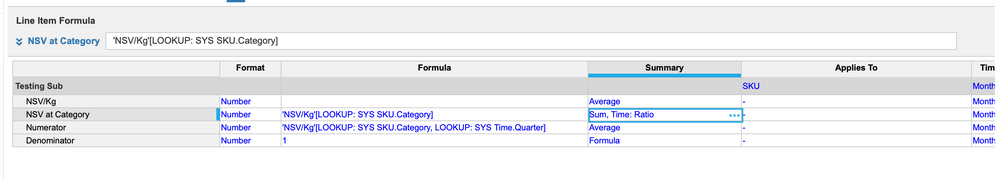Thanks,

Misbah
Miz Logix

•I see the problem! Did you try replicating the ratio summary in the staging line item?

Set the main summary as Average and only the Time summary as ratio. Then use the same ratio logic that you are using the NSV/KG line item? It will only apply to the time summary. Whatever line items are required for the ratio to work at the category level must also be looked up to the SKU level

Any way I can replicate this on my end? I don't understand how the ratio summary is working and that would be helpful

•Thank you @Misbah  @anirudh  both for your help. Your solution worked like a charm and I got the desired result on time dimension. But later one I realized that Average (for main summary won't do the work) - I forgot to mention there is a third dimension (Entity) that I'd like to apply same formula. Meaning that Average is allright for SKU (parents), but not allright for entity, where I need to apply same as time dimension (if that make any sense at all, it).

I did find a workaround to this, it's not helping at all with the sparcity, but I guess I cannot have both of them, can I?

- I created a new line item for each category and used [select:] in order to bring in the values, instead of lookup:

- then I created a final line item that summarizes the values with [ if conditions]. And of course this finally allowed me to use formula summary method, but again, it's a compromise I have to make, accuracy vs sparsity.  Maybe an additional step I can make is to create subsets for each of the category and only apply the line items to those subsets, it will drastically reduce some of the size.

@anirudh to come back to your question, the ration applied for NSV/KG is just a weighted average (NSV/KG*Volumes / total volumes).